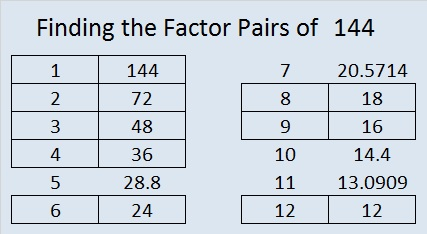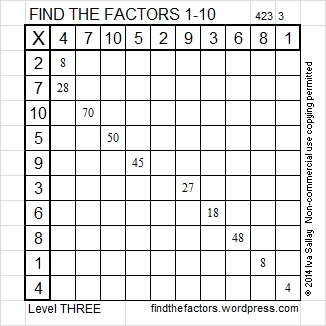# 144 and Level 3

144 is a composite number and a perfect square. Factor pairs: 144 = 1 x 144, 2 x 72, 3 x 48, 4 x 36, 6 x 24, 8 x 18, 9 x 16, 12 x 12. Factors of 144: 1, 2, 3, 4, 6, 8, 9, 12, 16, 18, 24, 36, 48, 72, 144. Prime factorization: 144 = 2 x 2 x 2 x 2 x 3 x 3, which can also be written 144 = (2^4) x (3^2).144 is the largest clue that appears in the FIND THE FACTORS 1 – 12 puzzles. For the puzzles in which it is a clue, always use 12 x 12 as the factors.

Excel file of puzzles and previous week’s factor solutions: 10 Factors 2014-06-09

A Logical Approach to FIND THE FACTORS: Find the column or row with two clues and find their common factor. Write the corresponding factors in the factor column (1st column) and factor row (top row).  Because this is a level three puzzle, you have now written a factor at the top of the factor column. Continue to work from the top of the factor column to the bottom, finding factors and filling in the factor column and the factor row one cell at a time as you go.This site uses Akismet to reduce spam. Learn how your comment data is processed.